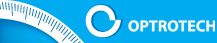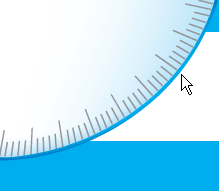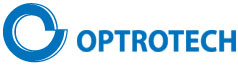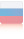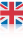OPTICAL DIGITAL MEASURING SYSTEMSCOMPANYPRODUCTSRESEARCHSERVICEPUBLICATIONPATENTSACHIEVEMENTPARTNERSCONTACTSRESEARCH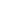Automated optical digital linearity deviation meterNew concept of angle measurement; Simulation and experimental studiesLinear and engular measurements using CCD camerasPrecision of image positioning by CCD cameraDigital Two-Dimensional Autocollimator with resolution 0.001” of engleCalibration of Digital Two-Dimensional AutocollimatorMetrological research and selection of target shape in digital measuring systemsLinewidth Measurement in Optical and Atomic-force Microscope for Metrological Control of PhotomasksMeasurement of diameter and geometry of precision ball bearingsMeasurement and correction of distortion in coordinate measurements by CCD cameraRESEARCH# New concept of angle measurement; Simulation and experimental studies

Korolev А.N.,  Lukin A.Ya.,  Polishchuk G.S.

Among great number of known precision converters and angle measuring instruments, optical-electronic devices with rasterization and code conversion are the most perfect ones. Those types of measuring instruments are produced in Russia (SKB IS ) as well as abroad (HEIDENHAIN GmbH (Germany)  and RENISHAW (Great Britain) ).

In such angular optoelectronic raster converters radial or cylindrical raster scale which is read by the analyzer made in a form of a raster as well is used as angular measures. For the precise convertors the amount of lines in the raster reaches few hundreds of thousands when the spacing between the lines equals to few micrometers. Therefore the dimensions of glass disks with raster scale are 20-30 sm. Due to the high requirements to stability of positional relationship of analyzer and measuring raster the convertor need to be mounted on the high precision spindle using precision or air pressure bearings.

For accuracy level of few seconds of arc and less the principal limitation of such devices are:

- large scale and weight;
- limitations of measurement accuracy due to backlash and vibration influence;
- high cost.

The objective of this paper is the description of new concept of angle measurements, based on measurement of rotation angle of a target (represented as an ordered set of single-type elements) image, relative to the rectangular coordinate system of rows and columns of digital camera photodetector , and also the analysis of some results of simulation and experimental study of new measurement technology.

Let us formulate the main distinct peculiarities of new angle measurement concept.

First of all, it is the transfer from analysis of 1D raster scale to analysis of 2D information field which requires brand-new design concepts and data processing technology. The important factor here is the high level of averaging of target elements coordinates that is proportional to M2, where M is the number of elements in the measuring field, since calculation of weight-average coordinates is performed for M elements along the X axis and for M elements along Y axis. The total number of elements in analyzed field can reach few hundreds.

Secondly, due to the high level of averaging, measuring system becomes extremely tolerable to such destabilizing factors as detector noise and etc. As a result the new measurement technology has high resolution that allow us to use small size measuring field. Therefore, the measurement error on the level of 1 second of arc can be obtained on the light-sensitive array with dimension less than 10 mm. Which is almost two orders smaller than dimension of raster sensors.

Thirdly, as a consequence of new technology there is no connection of angle measurement results to rotation axis. It is obvious that any transversaltranslational displacements will not change the results of angle measurement. This effect makes the new technology essentially different from well-known technologies described above.

Fourthly, it should be noted that new angle meter can be certified via standard with its further mounting on the measuring object which is impossible for raster convertors mentioned above.

In the fifth place, the possibility of target images synthesis with any random rotation angle with high precision allow us to obtain angle standards in the form of digital files. This is completely new case in metrology. But in a sense there is no contradiction in this. Because, unlike majority of measurands, angle, by definition, is dimensionless value.

All listed above characteristics and capabilities taken together allow us to speak about new conception of angle measurement that can serve as the basis for development of wide class of rather simple, compact and reasonably priced sensors and plane angle meters with different purposes and accuracy level.

It is worth mentioning that for this technology number of measurements per unit time is determined by frame frequency of TV camera. Therefore, in order to increase operation speed, high-speed TV cameras with high frame frequency should be used.

Fig. 1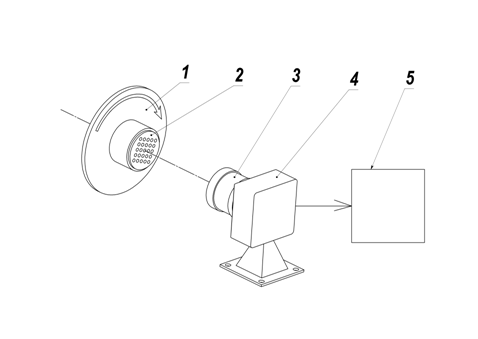Scheme of angle measurement device that illustrates the new conception is represented on the fig.1. Optical target 2 with the lamp rotates along with rotor 1 and is imaged by the lens 3 on the photodetector of digital camera 4 and then is send to the computer 5, which performs conversion and data processing, ensuring determination of target rotation angle relative to photodetector array.

The optical target configuration is a 2D set of elements with known positioning, for example, orthogonal grating composed of circle elements with relative brightness 1 on the background with relative brightness 0.

Due to the fact that orthogonal grating has rotation symmetry for certain angles (0, 90, 180 and 270 degrees), labels that uniquely determine target orientation are required. Three elements of the target that have larger diameter than other ones form scalene triangle. The position of this triangle is used for determination of grating orientation and ensures measurement range 0-360о.

It is obvious, that by increasing the number of elements in the array and dimensions of digital camera photodetector we can obtain the higher measurement accuracy. At the same time, element dimensions (the circle diameter) determines the accuracy of element coordinate determination and by decreasing it we decrease accuracy. The optimal configuration involves a certain compromise between elements diameter and array spacing.

It is obvious, that in current scheme target can be set even at considerable distance from rotor rotation axis.

In this paper we do not discuss sensor design, optimal parameters of the target and peculiar algorithms of video data processing and transformation that are designed to obtain the angle magnitude.

Fig.2 shows angular scale of experimental angle measuring instrument alongside with measurement result and software program panel with elements of data input and measurement process control, that include image of optical target. As a radiation detector we used TV camera BR-1340LM-UF  with 5.7 by 6.3 mm CMOS*) matrix (1280х1024 pixels, one pixel 5.2х5.2 mkm), lens magnification equals to 1.

Fig. 2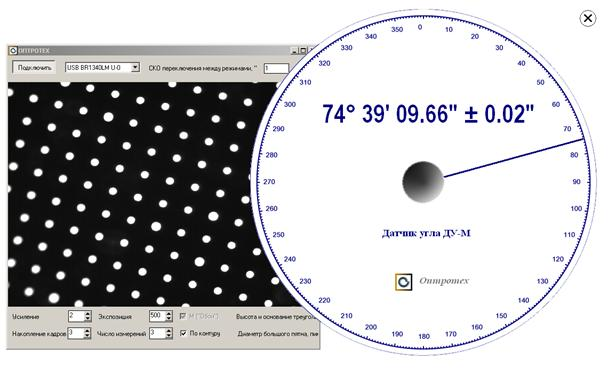On the bottom of program panel you can see input windows: “Accumulation of frames” – 3, “number of measurements” – 3. This means that for single measurement we need to carry out the accumulation of three frames. In order to receive the result presented on the dial plate we used three measurements. When more than one measurement is used we need to calculate the MSE of measurement result. The MSE is then shown on the dial plate near measured angle value as
“± 0.02"”. For multiple metering in the same angular position during about one minute, repeatability of measurements amounted to 0.08".

Quite a number of factors affect measurement result. However, the affect of such characteristics of detector as nonlinearity of light characteristic, dark noise, photon noise, irregularity of pixels sensitivity, charge spreading (blooming) and etc. can be minimized with the help of different algorithmic tools.

In this case, main factors that determine measurement error are quantization noises and anisotropic distortion of the lens, which lead to the deterministic shifts of some elements of the target. Since sampled digital image of the target is a picture of overlap of two periodic structures (periodic structure of target elements and structure of light sensitive array), resonance beat patterns appear in certain positions that lead to elements displacements and tempering of measurement results. These deterministic shifts make essential contribution to the measurement error and even can give isolated spikes out of 3σ, where σ is MSE of random deterministic measurement error in the measurement range 0-360о. These spikes can be reduced by implementation of stochastic deviation of target geometry with regard to elements diameter and spacing between them. This variant was successfully tested during model studies.

Peak value of lens distortion in the experimental prototype of angle measurer on the edge amounted to just few microns. For the target with periodic structure of elements precise measurement of lens distortion can be easily conducted. Such magnitude of distortion lead to the measurement constant error in each point of angular scale, and its peak value can reach 10-15 seconds of arc. Use of optics with higher quality can significantly reduce this error fraction, and in the case of zero distortion exclude it at all.

Metrological certification of experimental prototype of angle meter was conducted using Measuring Rotary table (MRT) which uses raster method of angle measurement and holographic angular scale with raster spacing about 1 mkm and diameter 180 mm. MRT was made in the Laboratory of informational-measuring systems of B.P.Konstantinov Petersburg Nuclear Physics Institute RAS . This device is used in the Laboratory of angular measurements D.I. Mendeleev Russian National Research Institute of Metrology as a part of National Angle Standard, its estimated accuracy is about 0.2 seconds of arc.

At first we conducted the calibration of angle-meter in the range 0-360о in increments of 1 degree. Received set of points describes constant error of angle-meter, associated with distortion. This smooth enough function is carried into the software memory and allows to implement correction in any point of the scale. Cubic interpolation is conducted in order to calculate the correction value inside 1 degree intervals.

Fig. 3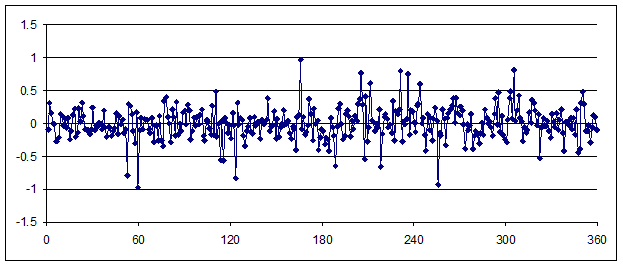Graph 3 represents the deviation of readings of experimental prototype of angle meter from mentioned above standard angle measuring device in the range 0-360 degrees with correction of constant error taken into account. Standard deviation of single measurement error is 0.25", peak deviation is about 1".

It is rather complex to experimentally determine optimal target configurations and limiting metrological capabilities of this new measurement technology during the study.

Therefore it became obvious, that it is necessary to develop mathematical model of angle measurement device with target image generator for any possible rotation angle and standard for such meter algorithm of target image processing and angle calculation. The development of software for target image generation is interesting also in connection with the possibility of developing the digital angle standards for this technology in the form of image files.

For the correct and complete description of target image in the simulation program, generator should take into account the following parameters: 1) size of photodetector matrix in pixels, 2) diameter of target elements, 3) target array spacing, 4) lens modulation transfer function, 5) noises of photodetector matrix, 6) number of frames used for averaging of results for single measurement, 7) number of measurements used for averaging in order to receive final measurement result.

The influence of the distortion was not taken into account in this model.

The main problem of developing a new model was the generation of sampled image of the target for arbitrary angle. It is rather difficult to generate such data array based on the analytical description of the array elements with the help of bit-by-bit integration. Therefore we applied, used in the work , method of generation of sampled target elements with fractional center of gravityshift relative to the array of readings.

Let the x0n,k, y0n,k be the coordinates of circular aperture centers along gratings rows n and columns k for rotation angle α = 0. Center coordinates after rotation by the arbitrary angle α were calculated according to well-known formula of coordinates transformation: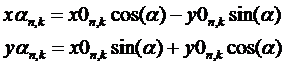(1).

After division of center coordinates by pixel size of specific CCD (CMOP) matrix we obtain coordinates values in fractions of pixel

After division of center coordinatesn,k  and  n,k  of the element with number n,k by pixel size of CMOP matrix we obtain coordinates values in fractions of pixel. The integer part of received values determines the position of this element in the sampled image of the target, while the fractional part is used to synthesize the image of this element with the center of gravity shift by the fraction of a pixel.

Synthesis of discrete model of each target circular aperture consisted of the following operations:

1. Calculation of object's Fourier spectrum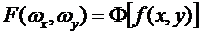,  (3)

where ω is the spatial frequency, Ф is direct Fourier transform. Object f(x,y) is a circle of chosen diameter with relative brightness 1 on the background with relative brightness 0.

2. Calculation of the image spectrum, which was shifted and blurred by the lens, according to the following formula: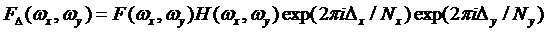, (4)

where exponential factor is a phase shift, which ensures shift of the object by Δx and Δy that correspond to fractional part of the coordinates n,k and n,k, H(ωxy) is the lens modulation transfer function (MTF), calculated according to well-known formula for diffraction-limited system: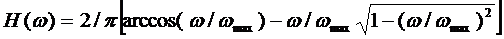, (5)

ωmax is cutoff spatial frequency of MTF.

3. Calculation of the digital array, which corresponds to the circular aperture image, shifted relative to the array of readings by a given value of Δx and Δy,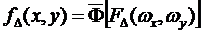,  (6)

where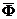is an inverse Fourier transform.

After quantization (f.e. 256 levels for 8 bit per pixel) and normalization the obtained image of each element is inserted into corresponding area of digital data array, that describes the complete picture of target discrete image.

It is worth mentioning that inaccuracies of production (generation) of target itself as well as limited number of quantization levels is the reason of coordinate error in the form of arbitrary shift of each element relative to ideal array of target elements for both generated target image and target image received from digital camera in real-time. Hence follows the high requirement to the accuracy of target making and to the number of quantization levels and photodetector noises.

Discussed technology of target image generation for arbitrary rotation angle involve calculation of M direct and inverse Fourier transforms where M is the number of elements in the target. Simulation experiments for great number of elements which include target synthesis and its measurement are computationally intensive.

During this study we analyzed rather large amount of choices of combined parameters of optical target but we can hardly say that the absolutely optimal alternatives were found.

Developed model offers a possibility to investigate parameters of desired signal for threshold angular displacements of target image. If the measurement field is limited by the circle with diameter slightly less than matrix diagonal, i.e. 8 mm, and rotation center is in the middle of the matrix, extreme elements of the target while rotation to the 360о travel over distance equal to the perimeter of circle 8 π  = 25 mm. Since one complete angle contains 1296000 seconds of arc while rotation to the 1 second of arc image of extreme elements moves over a distance of 20 nm. And what happens with target image?

Let us take the difference between two modeling images of the target with angular displacement equals to 1 second of arc. Computation results show that on the edge of the elements (circular apertures) that have peak value of displacement for about 10-12 pixels we receive difference of 1 quantizing level, that is illustrated in the fig.4 on the magnified picture of absolute value of images difference with normalization on full brightness. It should be noted that on the one side of the elements (in the direction of rotation) we obtain change of +1 quantizing level and on the other side -1 quantizing level. The fragment presented on the fig. 4 correspond to the left upper corner of the target image; rotation axis direction approximately through the right down corner. In order to maintain experiment integrity image generation was conducted without noise overlap.

Fig. 4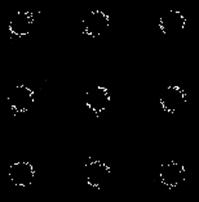We conducted three different variants of virtual measuring experiment with estimation of absolute measurement error as a difference between angle values calculated using target images synthesized by generator and rated values of target rotation angle for generation of its image; basic experiment parameters are presented in the table 1.

Table 1

 Experiment version Matrix size, pixels Pixel size, mkm Number of target elements Lens resolution, mm-1 Photodetector noise, quantizing levels Number of measurements 1 1280х1024 5.2 357 50 1 3 2 3000х2200 3.5 825 70 1 3 3 5000х2200 4.2 1971 70 1 3

The results of conducted virtual measuring experiments are presented in the table 2.

Table 2

 Experiment version MSE of measurement result error (σ) seconds of arc Maximum error value (3 σ) seconds of arc 1 0.18 0.53 2 0.04 0.12 3 0.007 0.022

Modeling results for the variant 1 are well correlated with the results of metrological certification of experimental angle meter. Modeling results for the variants 2 and 3, for which the matrix with significantly larger dimension were used, show that potential metrological possibilities of new concept of angle measurement allow us to speak about accuracy level of hundredths of second of arc.

Conclusions:

The results of experimental and simulation studies of estimation of metrological characteristic of the angle meter designed according to the new conception of angle measurement were described. The concept was developed by the authors of the paper and is based on the determination of angular position of image of optical target on the image sensor of TV camera using special data processing algorithms. The mathematical model of angle meter that uses target images generator with any rotation angle in rectangular coordinates and standard for this angle-meter data processing algorithm was developed. Metrological study of experimental prototype showed that error of angle-meter does not exceed 1 second of arc. Simulation study showed that potential accuracy level of new technology of angle measurement is hundredths of second of arc.

* CMOS Complementary-symmetry/metal-oxide-semiconductor.

References

1.  Booklet of SKB IS company www.skbis.ru),

2.  Booklet of HEIDENHAIN GmbH company (www.heidenhain.de)

3. Booklet of RENISHAW company (www.renishaw.com)

4. Korolev A.N., Lukin A.Ya., Polishchuk G.S., the Patent RU № 109847 «Angle-Meter».

5. Booklet of  ES-Experts» company (www.es-experts.ru)

6. S.V.Gordeev, B.G.Turukhano, Investigation of the interference field of two spherical waves for holographic recoding of precision radial diffraction gratings, Optics & Laser Technology, vol. 28, № 4, p. 255-261.

7. Korolev A.N., Gartsuev A.I., Polishchuk G.S., Tregub V.P. Metrological studies and the choice of the shape of an optical mark in digital measuring systems. Journal of Optical Technology. Vol. 77 Issue 6. pp.370-372 (2010).